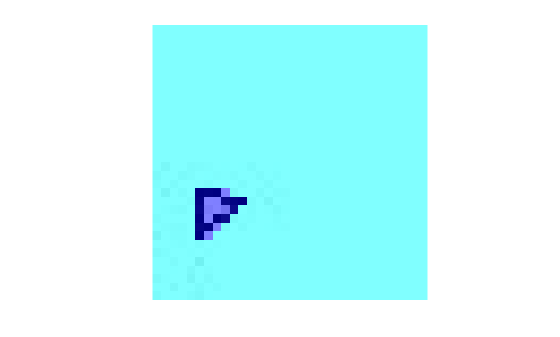# segmentationConfusionMatrix

Confusion matrix of multi-class pixel-level image segmentation

Since R2020b

## Syntax

``confusionMatrix = segmentationConfusionMatrix(LPred,LTruth)``

## Description

example

````confusionMatrix = segmentationConfusionMatrix(LPred,LTruth)` computes a confusion matrix from the predicted pixel labels `LPred` and ground truth pixel labels `LTruth`.```

## Examples

collapse all

Load a pretrained network that performs binary segmentation of triangles against a background.

`load('triangleSegmentationNetwork');`

The `triangleImages` data set has 100 test images with ground truth labels. Define the location of the data set.

`dataSetDir = fullfile(toolboxdir('vision'),'visiondata','triangleImages');`

Get one test image.

`testImage = imread(fullfile(dataSetDir,'testImages','image_001.jpg'));`

Get the corresponding ground truth label. The label image is stored as a numeric image, so convert the ground truth label to a categorical image.

```gtLabel = imread(fullfile(dataSetDir,'testLabels','labeled_image_001.png')); classNames = ["triangle","background"]; labelIDs = [255 0]; gtLabel = categorical(gtLabel,labelIDs,classNames);```

Display the ground truth labels over the test image.

`imshow(labeloverlay(testImage,gtLabel))`Perform semantic image segmentation.

`predLabel = semanticseg(testImage,net);`

Get the confusion matrix of the predicted labels and ground truth labels. The confusion matrix shows the segmentation correctly classified 21 pixels as triangle and 939 pixels as background. The confusion matrix also shows that the segmentation misclassified 64 background pixels as triangle. No triangle pixels are misclassified as background.

`confusionMatrix = segmentationConfusionMatrix(predLabel,gtLabel)`
```confusionMatrix = 2×2 21 0 64 939 ```

To check the accuracy of the confusion matrix, display the predicted labels over the test image. The overlay agrees with the values in the confusion matrix.

`imshow(labeloverlay(testImage,predLabel))`## Input Arguments

collapse all

Predicted pixel labels after semantic segmentation, specified as a 2-D label image or 3-D label image.

Data Types: `double` | `logical` | `categorical`

Ground truth pixel labels, specified as a 2-D label image or 3-D label image of the same size as the predicted pixel labels, `LPred`.

Data Types: `double` | `logical` | `categorical`

## Output Arguments

collapse all

Confusion matrix for the classes in the segmented images, returned as a C-by-C numeric matrix, where C is the number of classes in the semantic segmentation. Element (i,j) is the count of pixels known to belong to class i but predicted to belong to class j.

## Tips

• You can calculate semantic segmentation metrics such as the Jaccard score and classification accuracy from the confusion matrix by using the `evaluateSemanticSegmentation` function.

• You can use this function in block-based image processing workflows such as `bigimage`. For example, you can perform semantic segmentation and calculate the confusion matrix for a block in the same call to the `apply` function.

## Version History

Introduced in R2020b